Courses

# Time And Distance MCQ 2

## 20 Questions MCQ Test Quantitative Techniques for CLAT | Time And Distance MCQ 2

Description
This mock test of Time And Distance MCQ 2 for Quant helps you for every Quant entrance exam. This contains 20 Multiple Choice Questions for Quant Time And Distance MCQ 2 (mcq) to study with solutions a complete question bank. The solved questions answers in this Time And Distance MCQ 2 quiz give you a good mix of easy questions and tough questions. Quant students definitely take this Time And Distance MCQ 2 exercise for a better result in the exam. You can find other Time And Distance MCQ 2 extra questions, long questions & short questions for Quant on EduRev as well by searching above.
QUESTION: 1

Solution:
QUESTION: 2

Solution:
QUESTION: 3

### A student walks from his house at 2 km/h and reaches school 5 minutes late and if his speed had been 3 km/h he would have reached the school 5 minutes earlier. How far is his school from house?

Solution:

Speed = Distance/Time
Let ideal time taken to reach school is t, distance to school = distance(d)
With a speed of 4km/hr or 4/60 km/min he reaches 5 mins late i.e.
4/60= d/(t+5)
60d = 4t + 20 ----- (1)
Similarly
5/60 = d/(t-10)
60d = 5t - 50 ----- (2)
From eq 1 and 2
4t +20 = 5t - 50
t = 70 mins
60d = 4t + 20 = 280 + 20 = 300
distance(d) = 5 kms

QUESTION: 4

A person walks from his house at the rate of 6 km/h and reaches office 12 minutes late and if his speed had been 8 km/h he would have reached the office 8 minutes earlier. How far is his office from house?

Solution:
QUESTION: 5

By walking 3/2 of his usual speed a person reach his office 10 minutes earlier. Find his usual time.

Solution:
QUESTION: 6

Byof  2/5 his usual speed a student reach his school 30 minutes late. Find his usual time?

Solution:
QUESTION: 7

A train whose length is 300m long is moving at the rate of 108 km/h. How long will it take to pass a pole?

Solution:
QUESTION: 8

How long does a train 200 m long moving at the rate of 72 km/h take to pass a tunnel 40 m long?

Solution:
QUESTION: 9

How long does a train 600 m long moving at the rate of 144 km/h take to pass a bridge 200 m long?

Solution:
QUESTION: 10

Two trains of 400 m and 350 m long are running on parallel tracks at the rate of 180 km/h and 120 km/h respectively. How long will they take to cross each other if they are running in opposite direction?

Solution:
QUESTION: 11

Two trains 500 m and 200 m long are running on parallel tracks at the rate of 160 km/h and 200 km/h. How long the second train take to cross 1st train if they are running in same direction.

Solution:
QUESTION: 12

A train crosses a platform of length 350 m in 12 sec and a man standing on platform in 2 sec. Find the speed of train.

Solution:

The correct answer is B as
Let the length of train be x m.
Since the train crosses the man in 2 sec
Speed of train = x/2 m/s
Also it crosses the platform of length 350 m in 12 sec
So speed of train = (350+x)/12
Equating both the speeds of train ,we get
x/2 = (350+x)/12
x = (350+x)/6
6x = 350+x
5x = 350
x = 70
Thus length of train = 70 m
Now, speed of train = 70/2 = 35 m/s
= 35/1000*3600 = 35/5*18
=126 km/hr.

QUESTION: 13

A man moves downstream at a rate of 14 km/h and upstream at a rate of 6 km/h. Find his speed in still water.

Solution:
QUESTION: 14

A man moves downstream at a rate of 14 km/h and upstream at a rate of 6 km/h. Find his speed in still water.

Solution:
QUESTION: 15

A boat moves down the stream at the rate of 20 km/h and it takes 12 minutes for him to move one km against it. Find the speed of stream.

Solution:
QUESTION: 16

A man can swim 4 km/h in still water. If the velocity of stream is 2 km/h, the time taken by him to swim to a place 12 km upstream and back is:

Solution:
QUESTION: 17

A man can row three quarter of a km against the stream in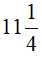min. and return in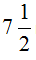min. The speed of man in still water is.

Solution:

Downstream = (3/4) (15/2)km/min = 1/10 =6km/hr
upstream = (3/4) ( 45/4) km/min =1/15 =4km/he
Stillwater = 1/2(6+4)= 5 km/hr

QUESTION: 18

A man takes twice as long to row a distance against the stream as to row the same distance in favour of the stream. The ratio of the speed of the boat (in still water) and the stream is:

Solution:

Let speed upstream = x
Then, speed downstream = 2x

Speed in still water =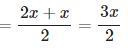Speed of the stream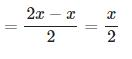Speed in still water : Speed of the stream  =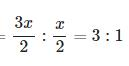QUESTION: 19

The speed of a boat upstream is 10 km/h. The speed of stream is 5 km/h. The speed of boatdown stream is.

Solution:
QUESTION: 20

A thief steals a car at 9 am and drive at the rate of 20 km/h. The theft is discovered and police starts chasing him at 9.30 am. in a jeep at the speed of 40 km/h. The thief will be caught at ………

Solution:

The  correct answer is A as
Relative speed = 40-20 (same distance)
Distance = 10 KM
Time = distance/time = 10/20 = 1/2 hours = 30 minutes
9.30 + 30 = 10 AM